# FeenoX annotated examples

All the files needed to run theses examples are available in the examples directory of the Git repository and any of the tarballs, both source and binary.

For a presentation of these examples, see the “FeenoX Overview Presentation” (August 2021).

Also, the tests directory in the Github repository has dozens of test cases which can be used as examples for reference and for mathematical verification of the results obtained with FeenoX.

# 1 Hello World (and Universe)!

PRINT "Hello $1!" $ feenox hello.fee World
Hello World!
$feenox hello.fee Universe Hello Universe!$

# 2 Lorenz’ attractor (the one with the butterfly)

Solve

\begin{cases} \dot{x} &= \sigma \cdot (y - x) \\ \dot{y} &= x \cdot (r - z) - y \\ \dot{z} &= x y - b z \\ \end{cases}

for 0 < t < 40 with initial conditions

\begin{cases} x(0) = -11 \\ y(0) = -16 \\ z(0) = 22.5 \\ \end{cases}

and \sigma=10, r=28 and b=8/3, which are the classical parameters that generate the butterfly as presented by Edward Lorenz back in his seminal 1963 paper Deterministic non-periodic flow. This example’s input file ressembles the parameters, inital conditions and differential equations of the problem as naturally as possible with an ASCII file.

PHASE_SPACE x y z     # Lorenz attractor’s phase space is x-y-z
end_time = 40         # we go from t=0 to 40 non-dimensional units

sigma = 10            # the original parameters from the 1963 paper
r = 28
b = 8/3

x_0 = -11             # initial conditions
y_0 = -16
z_0 = 22.5

# the dynamical system's equations written as naturally as possible
x_dot = sigma*(y - x)
y_dot = x*(r - z) - y
z_dot = x*y - b*z

PRINT t x y z        # four-column plain-ASCII output
$feenox lorenz.fee > lorenz.dat$ gnuplot lorenz.gp
$python3 lorenz.py$ sh lorenz2x3d.sh < lorenz.dat > lorenz.html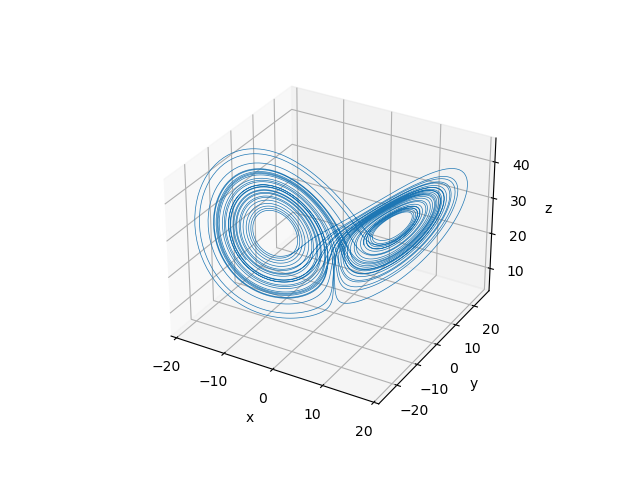Figure 1: The Lorenz attractor computed with FeenoX plotted with two different tools. a — Gnuplot, b — Matplotlib

# 3 The logistic map

Plot the asymptotic behavior of the logistic map

x_{n+1} = r \cdot x \cdot (1-x)

for a range of the parameter r.

DEFAULT_ARGUMENT_VALUE 1 2.6   # by default show r in [2.6:4]
DEFAULT_ARGUMENT_VALUE 2 4

steps_per_r = 2^10
steps_asymptotic = 2^8
steps_for_r = 2^10

static_steps = steps_for_r*steps_per_r

# change r every steps_per_r steps
IF mod(step_static,steps_per_r)=1
r = quasi_random($1,$2)
ENDIF

x_init = 0.5           # start at x = 0.5
x = r*x*(1-x)          # apply the map

IF step_static-steps_per_r*floor(step_static/steps_per_r)>(steps_per_r-steps_asymptotic)
# write the asymptotic behavior only
PRINT r x
ENDIF
$gnuplot gnuplot> plot "< feenox logistic.fee" w p pt 50 ps 0.02 gnuplot> quit$

# 4 Thermal slabs

## 4.1 One-dimensional linear

Solve heat conduction on the slab x \in [0:1] with boundary conditions

\begin{cases} T(0) = 0 & \text{(left)} \\ T(1) = 1 & \text{(right)} \\ \end{cases}

and uniform conductivity. Compute T\left(\frac{1}{2}\right).

• The input written in a self-evident English-like dialect
• Syntactic sugared plain-text ASCII file
• Simple problems (like this one) need simple inputs
• FeenoX follows the UNIX rule of simplicity
• Output is 100% user-defined
• No PRINT no output
• Feenox follows the UNIX rule of silence
• There is no node at x=1/2=0.5!
• FeenoX knows how to interpolate
• Mesh separated from problem
• The geometry comes from a Git-friendly .geo
Point(1) = {0, 0, 0};          // geometry:
Point(2) = {1, 0, 0};          // two points
Line(1) = {1, 2};              // and a line connecting them!

Physical Point("left") = {1};  // groups for BCs and materials
Physical Point("right") = {2};
Physical Line("bulk") = {1};   // needed due to how Gmsh works

Mesh.MeshSizeMax = 1/3;        // mesh size, three line elements
Mesh.MeshSizeMin = Mesh.MeshSizeMax;
• UNIX rule of composition
• The actual input file is a Git-friendly .fee
PROBLEM thermal 1D    # tell FeenoX what we want to solve
k = 1                 # set uniform conductivity
BC left  T=0          # set fixed temperatures as BCs
BC right T=1          # "left" and "right" are defined in the mesh
SOLVE_PROBLEM         # we are ready to solve the problem
PRINT T(1/2)          # ask for the temperature at x=1/2
$gmsh -1 slab.geo [...] Info : 4 nodes 5 elements Info : Writing 'slab.msh'... [...]$ feenox thermal-1d-dirichlet-uniform-k.fee
0.5
$ # 5 NAFEMS LE10 “Thick plate pressure” benchmarkThe NAFEMS LE10 problem statement and the corresponding FeenoX input Assuming the CAD has already been created in STEP format (for instance using Gmsh with this geo file), create a tetrahedral locally-refined unstructured grid with Gmsh using the following .geo file: // NAFEMS LE10 benchmark unstructured locally-refined tetrahedral mesh Merge "nafems-le10.step"; // load the CAD // define physical names from the geometrical entity ids Physical Surface("upper") = {7}; Physical Surface("DCD'C'") = {1}; Physical Surface("ABA'B'") = {3}; Physical Surface("BCB'C'") = {4, 5}; Physical Curve("midplane") = {14}; Physical Volume("bulk") = {1}; // meshing settings, read Gmsh' manual for further reference Mesh.ElementOrder = 2; // use second-order tetrahedra Mesh.Algorithm = 6; // 2D mesh algorithm: 6: Frontal Delaunay Mesh.Algorithm3D = 10; // 3D mesh algorithm: 10: HXT Mesh.Optimize = 1; // Optimize the mesh Mesh.HighOrderOptimize = 1; // Optimize high-order meshes? 2: elastic+optimization Mesh.MeshSizeMax = 80; // main element size Mesh.MeshSizeMin = 20; // refined element size // local refinement around the point D (entity 4) Field = Distance; Field.NodesList = {4}; Field = Threshold; Field.IField = 1; Field.LcMin = Mesh.MeshSizeMin; Field.LcMax = Mesh.MeshSizeMax; Field.DistMin = 2 * Mesh.MeshSizeMax; Field.DistMax = 6 * Mesh.MeshSizeMax; Background Field = {2}; and then use this pretty-straightforward input file that has a one-to-one correspondence with the original problem formulation from 1990: # NAFEMS Benchmark LE-10: thick plate pressure PROBLEM mechanical 3D READ_MESH nafems-le10.msh # mesh in millimeters # LOADING: uniform normal pressure on the upper surface BC upper p=1 # 1 Mpa # BOUNDARY CONDITIONS: BC DCD'C' v=0 # Face DCD'C' zero y-displacement BC ABA'B' u=0 # Face ABA'B' zero x-displacement BC BCB'C' u=0 v=0 # Face BCB'C' x and y displ. fixed BC midplane w=0 # z displacements fixed along mid-plane # MATERIAL PROPERTIES: isotropic single-material properties E = 210e3 # Young modulus in MPa nu = 0.3 # Poisson's ratio SOLVE_PROBLEM # solve! # print the direct stress y at D (and nothing more) PRINT "sigma_y @ D = " sigmay(2000,0,300) "MPa" # write post-processing data for paraview WRITE_MESH nafems-le10.vtk sigmay VECTOR u v w $ gmsh -3 nafems-le10.geo
[...]
$feenox nafems-le10.fee sigma_y @ D = -5.38016 MPa$Normal stress \sigma_y refined around point D over 5,000x-warped displacements for LE10 created with Paraview

# 6 NAFEMS LE11 “Solid Cylinder/Taper/Sphere-Temperature” benchmark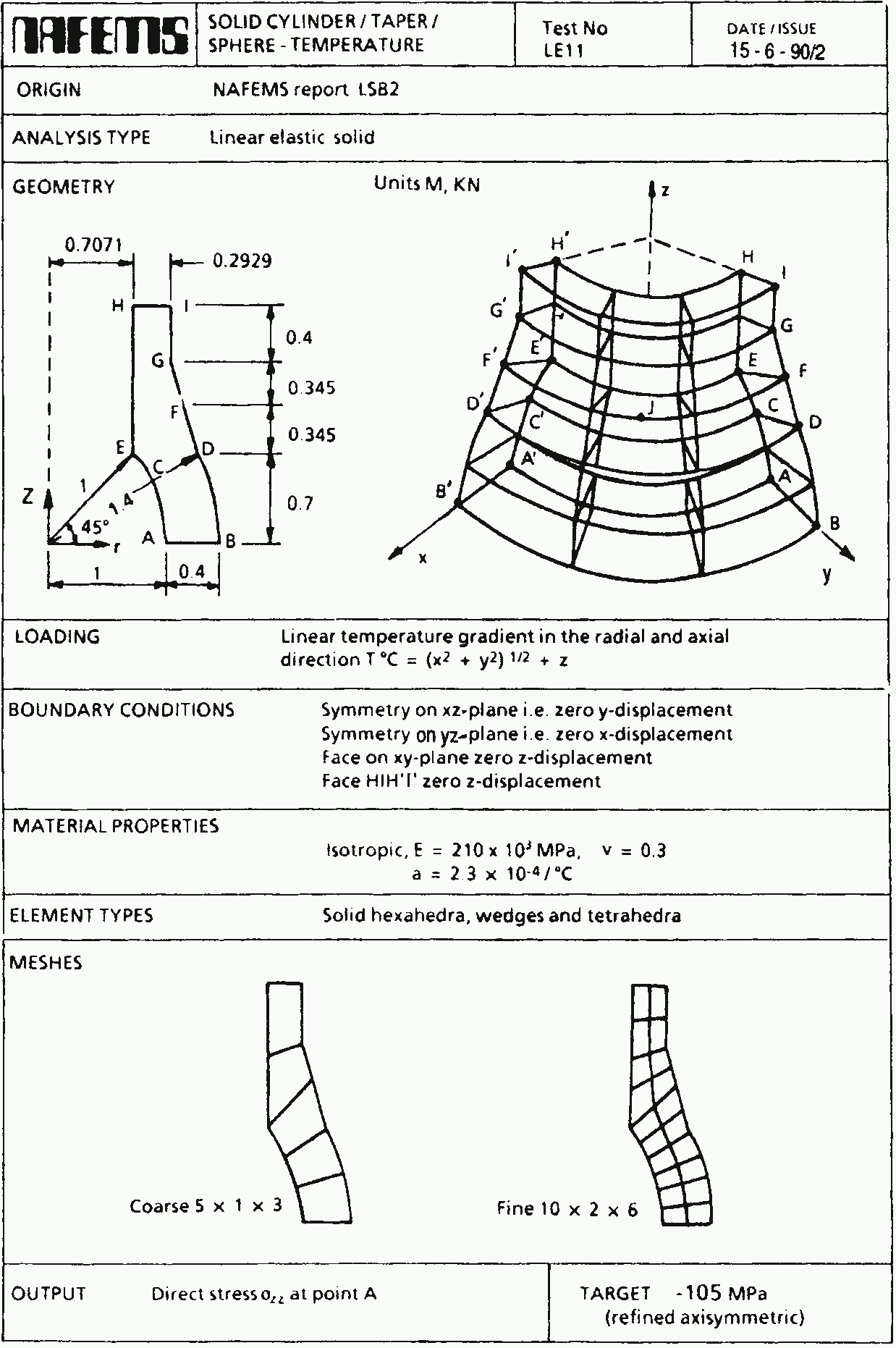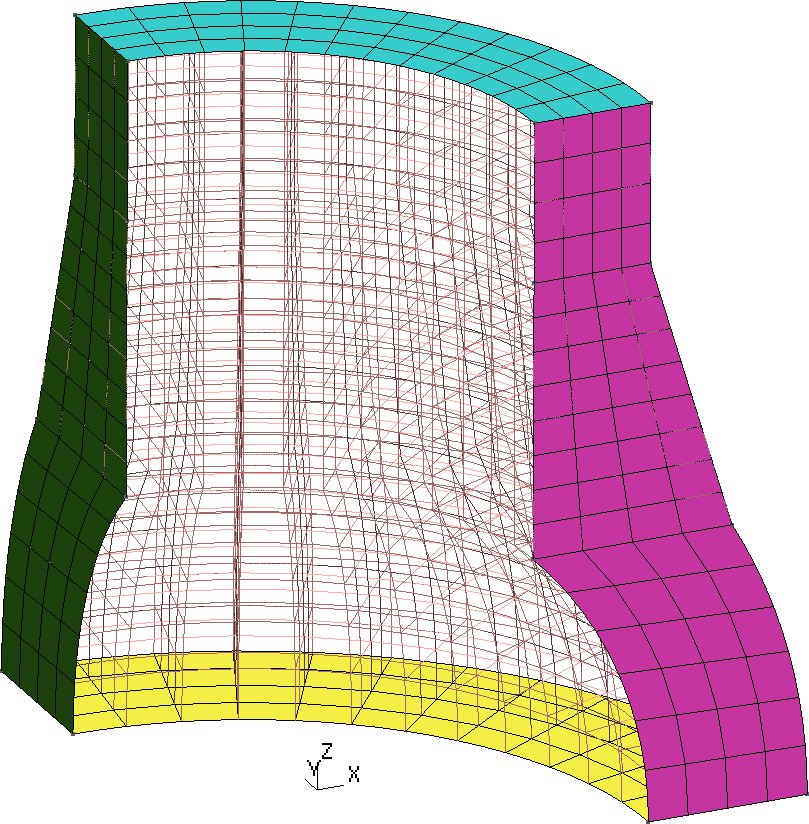Figure 2: The NAFEMS LE11 problem formulation. a — Problem statement, b — Structured hex mesh

Following the spirit from LE10, note how easy it is to give a space-dependent temperature field in FeenoX. Just write \sqrt{x^2+y^2}+z like sqrt(x^2 + y^2) + z!

# NAFEMS Benchmark LE-11: solid cylinder/taper/sphere-temperature
PROBLEM mechanical 3D

# as an algebraic expression as human-friendly as it can be
T(x,y,z) := sqrt(x^2 + y^2) + z

BC xz     v=0       # displacement vector is [u,v,w]
BC yz     u=0       # u = displacement in x
BC xy     w=0       # v = displacement in y
BC HIH'I' w=0       # w = displacement in z

E = 210e3*1e6       # mesh is in meters, so E=210e3 MPa -> Pa
nu = 0.3            # dimensionless
alpha = 2.3e-4      # in 1/ºC as in the problem
SOLVE_PROBLEM

# for post-processing in Paraview
WRITE_MESH nafems-le11.vtk VECTOR u v w   T sigmax sigmay sigmaz

PRINT "sigma_z(A) =" %.2f sigmaz(1,0,0)/1e6 "MPa" SEP " "
PRINT "wall time  =" %.2f wall_time() "seconds"  SEP " "
$gmsh -3 nafems-le11.geo [...]$ feenox nafems-le11.fee
sigma_z(A) = -105.04 MPa
wall time  = 3.24 seconds
$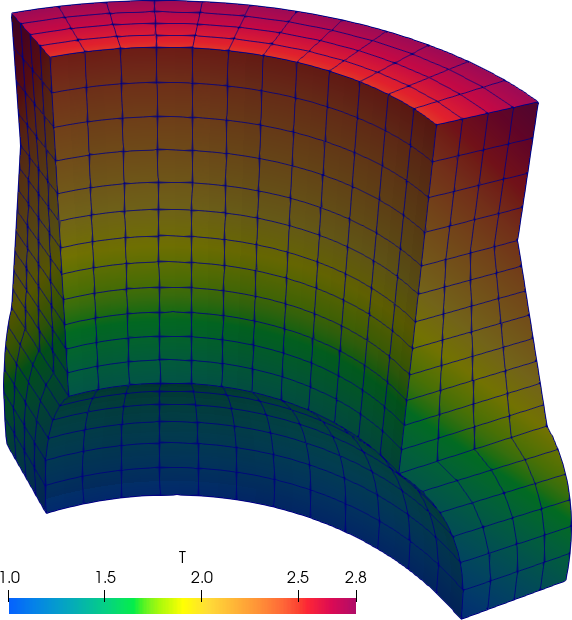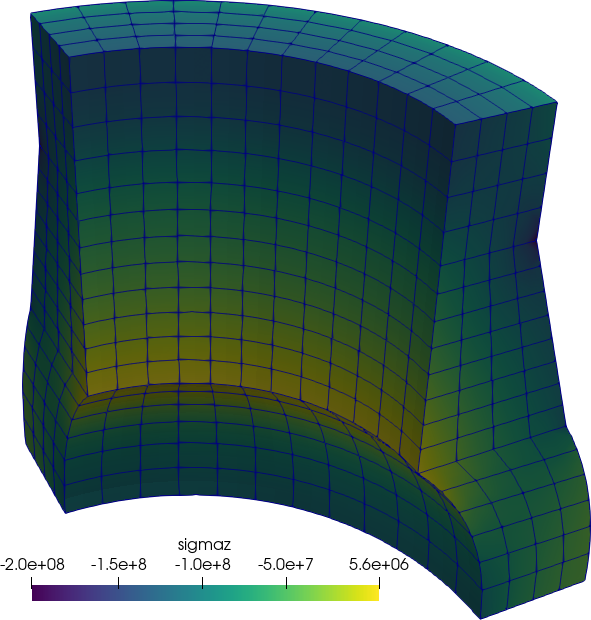Figure 3: The NAFEMS LE11 problem results. a — Problem statement, b — Structured hex mesh # 7 NAFEMS LE1 “Elliptical membrane” plane-stress benchmarkThe NAFEMS LE1 problem Tell FenooX the problem is plane_stress. Use the nafems-le1.geo file provided to create the mesh. Read it with READ_MESH, set material properties, BCs and SOLVE_PROBLEM! PROBLEM mechanical plane_stress READ_MESH nafems-le1.msh E = 210e3 nu = 0.3 BC AB u=0 BC CD v=0 BC BC tension=10 SOLVE_PROBLEM WRITE_MESH nafems-le1.vtk VECTOR u v 0 sigmax sigmay tauxy PRINT "σy at point D = " %.4f sigmay(2000,0) "(reference is 92.7)" SEP " " $ gmsh -2 nafems-le11.geo
[...]
$feenox nafems-le1.fee σy at point D = 92.7011 (reference is 92.7)$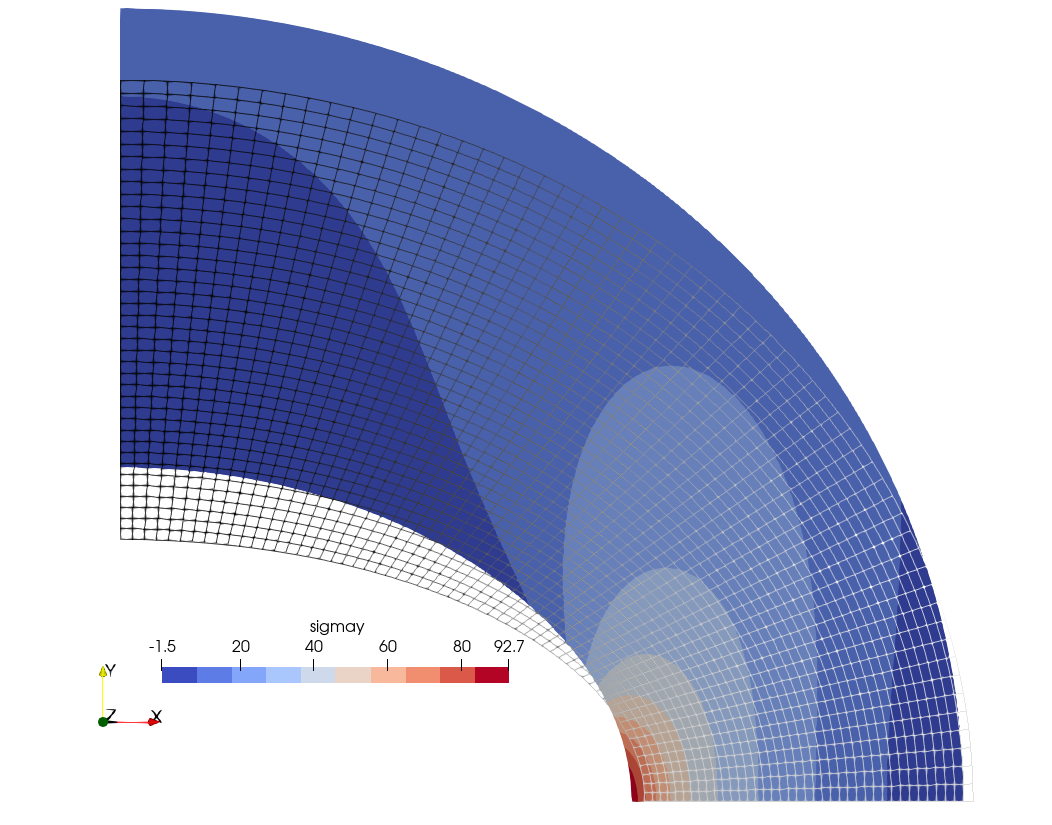Normal stress \sigma_y over 500x-warped displacements for LE1 created with Paraview

# 8 How to solve a maze without AI

Say you are Homer Simpson and you want to solve a maze drawn in a restaurant’s placemat, one where both the start and end are known beforehand. In order to avoid falling into the alligator’s mouth, you can exploit the ellipticity of the Laplacian operator to solve any maze (even a hand-drawn one) without needing any fancy AI or ML algorithm. Just FeenoX and a bunch of standard open source tools to convert a bitmapped picture of the maze into an unstructured mesh.

PROBLEM laplace 2D  # pretty self-descriptive, isn't it?

# boundary conditions (default is homogeneous Neumann)
BC start  phi=0
BC end    phi=1

SOLVE_PROBLEM

# write the norm of gradient as a scalar field
# and the gradient as a 2d vector into a .msh file
WRITE_MESH maze-solved.msh \
sqrt(dphidx(x,y)^2+dphidy(x,y)^2) \
VECTOR dphidx dphidy 0 
$gmsh -2 maze.geo [...]$ feenox maze.fee
1	1
2	1
3	2
4	3
5	5
6	8
7	13
8	21
9	34
10	55
11	89
12	144
13	233
14	377
15	610
16	987
17	1597
18	2584
19	4181
20	6765
$ ## 9.3 Solving an iterative problem Finally, we print the sequence as an iterative problem and check that the three outputs are the same. static_steps = 20 #static_iterations = 1476 # limit of doubles IF step_static=1|step_static=2 f_n = 1 f_nminus1 = 1 f_nminus2 = 1 ELSE f_n = f_nminus1 + f_nminus2 f_nminus2 = f_nminus1 f_nminus1 = f_n ENDIF PRINT step_static f_n $ feenox fibo_iterative.fee > three
$diff one two$ diff two three
$ # 10 Computing the derivative of a function as a UNIX filter This example illustrates how well FeenoX integrates into the UNIX philosophy. Let’s say one has a function f(t) as an ASCII file with two columns and one wants to compute the derivative f'(t). Just pipe the function file into this example’s input file derivative.fee used as a filter. For example, this small input file f.fee writes the function of time provided in the first command-line argument from zero up to the second command-line argument: end_time =$2
PRINT t $1 $ feenox f.fee "sin(t)" 1
0       0
0.0625  0.0624593
0.125   0.124675
0.1875  0.186403
0.25    0.247404
0.3125  0.307439
0.375   0.366273
0.4375  0.423676
0.5     0.479426
0.5625  0.533303
0.625   0.585097
0.6875  0.634607
0.75    0.681639
0.8125  0.726009
0.875   0.767544
0.9375  0.806081
1       0.841471
$ Then we can pipe the output of this command to the derivative filter. Note that • The derivative.fee has the execution flag has on and a shebang line pointing to a global location of the FeenoX binary in /usr/local/bin e.g. after doing sudo make install. • The first argument of derivative.fee controls the time step. This is only important to control the number of output lines. It does not have anything to do with precision, since the derivative is computed using an adaptive centered numerical differentiation scheme using the GNU Scientific Library. • Before doing the actual differentiation, the input data is interpolated using a third-order monotonous scheme (also with GSL). • TL;DR: this is not just “current value minus last value divided time increment.” #!/usr/local/bin/feenox # read the function from stdin FUNCTION f(t) FILE - INTERPOLATION steffen # detect the domain range a = vecmin(vec_f_t) b = vecmax(vec_f_t) # time step from arguments (or default 10 steps) DEFAULT_ARGUMENT_VALUE 1 (b-a)/10 h =$1

# compute the derivative with a wrapper for gsl_deriv_central()
VAR t'
f'(t) = derivative(f(t'),t',t)

# write the result
PRINT_FUNCTION f' MIN a+0.5*h MAX b-0.5*h STEP h
$chmod +x derivative.sh$ feenox f.fee "sin(t)" 1 | ./derivative.fee 0.1 | tee f_prime.dat
0.05    0.998725
0.15    0.989041
0.25    0.968288
0.35    0.939643
0.45    0.900427
0.55    0.852504
0.65    0.796311
0.75    0.731216
0.85    0.66018
0.95    0.574296
$Numerical derivative as a UNIX filter and actual analytical result # 11 Parametric study on a cantilevered beam If an external loop successively calls FeenoX with extra command-line arguments, a parametric run is obtained. This file cantilever.fee fixes the face called “left” and sets a load in the negative z direction of a mesh called cantilever-$1-$2.msh, where $1 is the first argument after the inpt file and $2 the second one. The output is a single line containing the number of nodes of the mesh and the displacement in the vertical direction w(500,0,0) at the center of the cantilever’s free face. The following Bash script first calls Gmsh to create the meshes. To do so, it first starts with a base cantilever.geo file that creates the CAD: // https://autofem.com/examples/determining_natural_frequencie.html SetFactory("OpenCASCADE"); L = 0.5; b = 0.05; h = 0.02; Box(1) = {0,-b/2,-h/2, L, b, h}; Physical Surface("left") = {1}; Physical Surface("right") = {2}; Physical Surface("top") = {4}; Physical Volume("bulk") = {1}; Transfinite Curve {1, 3, 5, 7} = 1/(Mesh.MeshSizeFactor*Mesh.ElementOrder) + 1; Transfinite Curve {2, 4, 6, 8} = 2/(Mesh.MeshSizeFactor*Mesh.ElementOrder) + 1; Transfinite Curve {9, 10, 11, 12} = 16/(Mesh.MeshSizeFactor*Mesh.ElementOrder) + 1; Transfinite Surface "*"; Transfinite Volume "*"; Then another .geo file is merged to build cantilever-${element}-${c}.msh where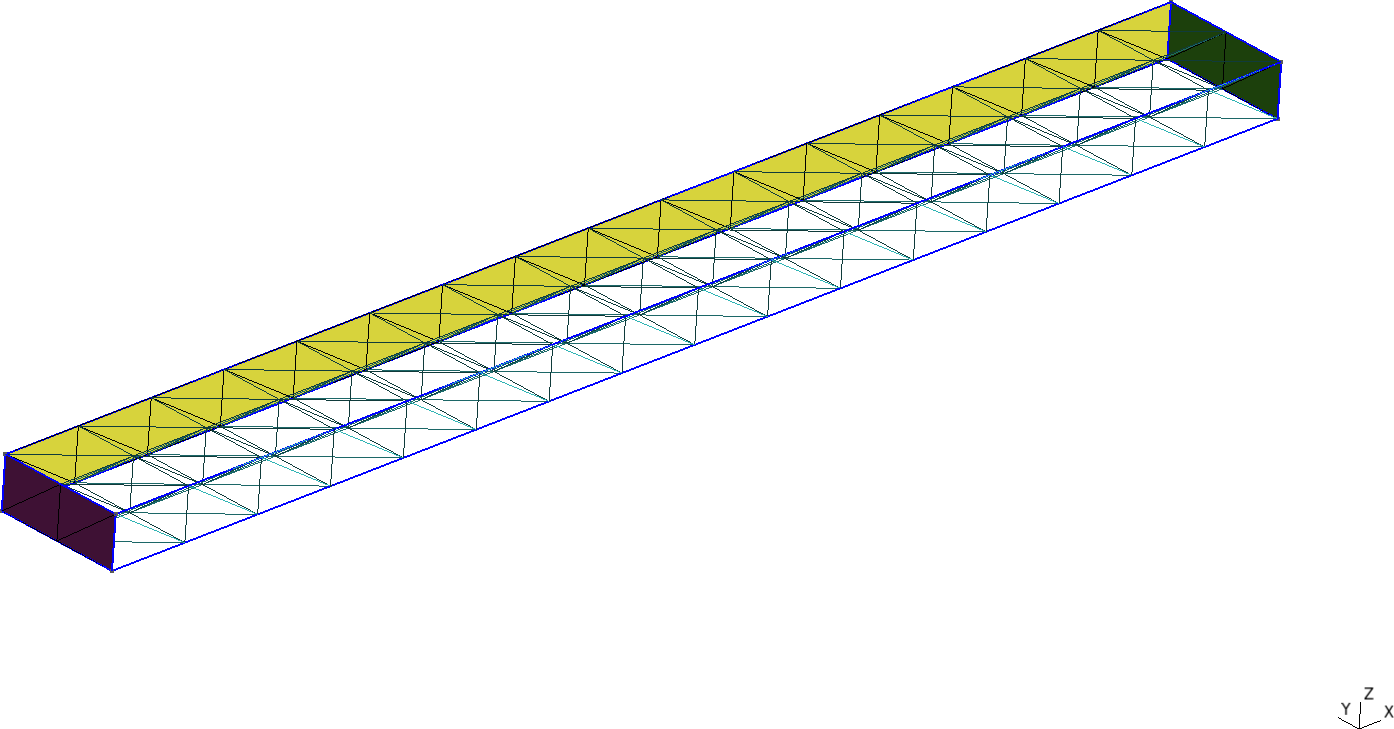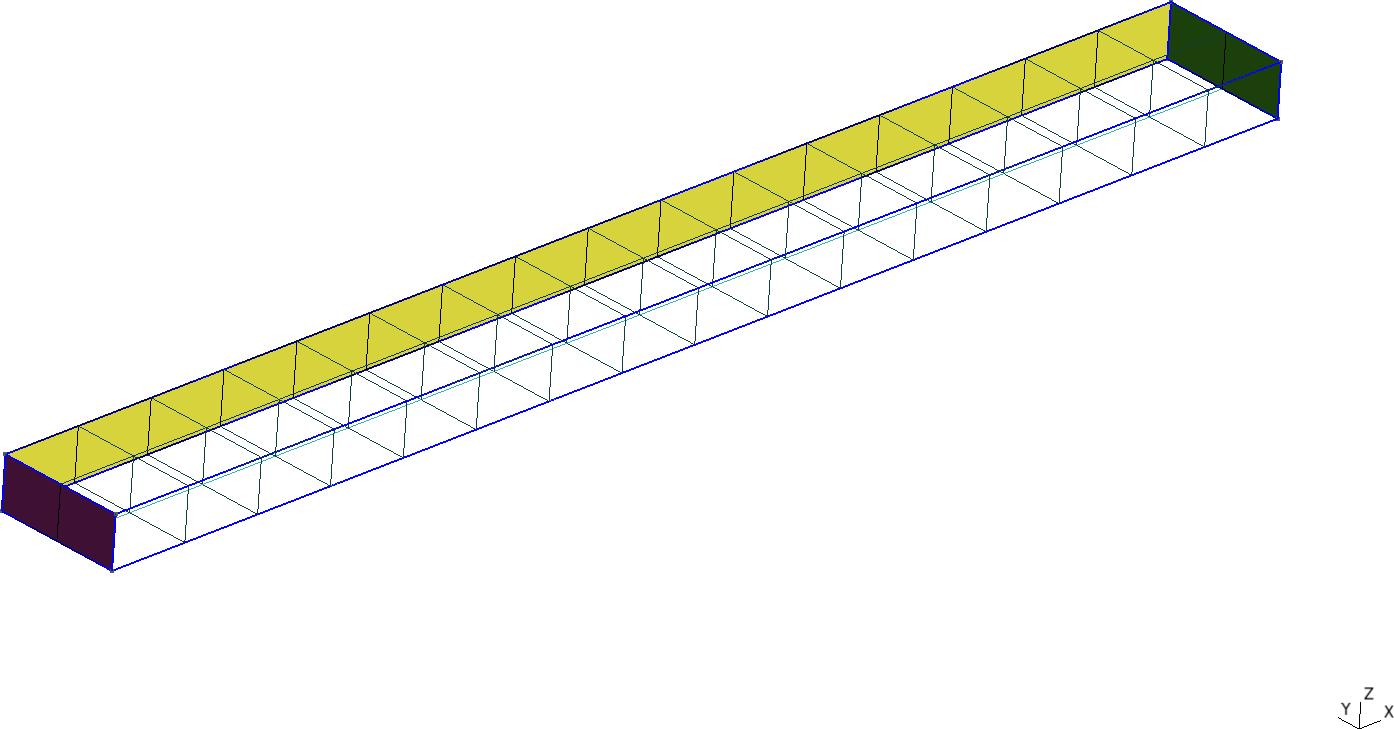Figure 4: Cantilevered beam meshed with structured tetrahedra and hexahedra. a — Tetrahedra, b — Hexahedra It then calls FeenoX with the input cantilever.fee and passes ${element} and ${c} as extra arguments, which then are expanded as $1 and $2 respectively. #!/bin/bash rm -f *.dat for element in tet4 tet10 hex8 hex20 hex27; do for c in$(seq 1 10); do

# create mesh if not alreay cached
mesh=cantilever-${element}-${c}
if [ ! -e ${mesh}.msh ]; then scale=$(echo "PRINT 1/${c}" | feenox -) gmsh -3 -v 0 cantilever-${element}.geo -clscale ${scale} -o${mesh}.msh
fi

# call FeenoX
feenox cantilever.fee ${element}${c} | tee -a cantilever-${element}.dat done done After the execution of the Bash script, thanks to the design decision that output is 100% defined by the user (in this case with the PRINT instruction), one has several files cantilever-${element}.dat files. When plotted, these show the shear locking effect of fully-integrated first-order elements. The theoretical Euler-Bernoulli result is just a reference as, among other things, it does not take into account the effect of the material’s Poisson’s ratio. Note that the abscissa shows the number of nodes, which are proportional to the number of degrees of freedom (i.e. the size of the problem matrix) and not the number of elements, which is irrelevant here and in most problems.

PROBLEM elastic 3D
READ_MESH cantilever-$1-$2.msh   # in meters

E = 2.1e11         # Young modulus in Pascals
nu = 0.3           # Poisson's ratio

BC left   fixed
BC right  tz=-1e5  # traction in Pascals, negative z

SOLVE_PROBLEM

# z-displacement (components are u,v,w) at the tip vs. number of nodes
PRINT nodes %e w(500,0,0) "\# $1$2"
$./cantilever.sh 102 -7.641572e-05 # tet4 1 495 -2.047389e-04 # tet4 2 1372 -3.149658e-04 # tet4 3 [...] 19737 -5.916234e-04 # hex27 8 24795 -5.916724e-04 # hex27 9 37191 -5.917163e-04 # hex27 10$ pyxplot cantilever.pplDisplacement at the free tip of a cantilevered beam vs. number of nodes for different element types

# 12 Optimizing the length of a tuning fork

To illustrate how to use FeenoX in an optimization loop, let us consider the problem of finding the length \ell_1 of a tuning fork (fig. 5) such that the fundamental frequency on a free-free oscillation is equal to the base A frequency at 440 Hz.Figure 5: What length \ell_1 is needed so the fork vibrates at 440 Hz?

The FeenoX input is extremely simple input file, since it has to solve the free-free mechanical modal problem (i.e. without any Dirichlet boundary condition). All it has to do is to print the fundamental frequency.

To find the length \ell_1, FeenoX is sucessively called from a Python driving script called fork.py. This script uses Gmsh’s Python API to create the CAD and the mesh of the tuning fork given the geometrical arguments r, w, \ell_1 and \ell_2. The parameter n controls the number of elements through the fork’s thickness. Here is the driving script without the CAD & mesh details (the full implementation of the function is available in the examples directory of the FeenoX distribution):

import math
import gmsh
import subprocess  # to call FeenoX and read back

def create_mesh(r, w, l1, l2, n):
gmsh.initialize()
...
gmsh.write("fork.msh")
gmsh.finalize()
return len(nodes)

def main():
target = 440    # target frequency
eps = 1e-2      # tolerance
r = 4.2e-3      # geometric parameters
w = 3e-3
l1 = 30e-3
l2 = 60e-3

for n in range(1,7):   # mesh refinement level
l1 = 60e-3              # restart l1 & error
error = 60
while abs(error) > eps:   # loop
l1 = l1 - 1e-4*error
# mesh with Gmsh Python API
nodes = create_mesh(r, w, l1, l2, n)
# call FeenoX and read scalar back
# TODO: FeenoX Python API (like Gmsh)
result = subprocess.run(['feenox', 'fork.fee'], stdout=subprocess.PIPE)
freq = float(result.stdout.decode('utf-8'))
error = target - freq

print(nodes, l1, freq)

Note that in this particular case, the FeenoX input files does not expand any command-line argument. The trick is that the mesh file fork.msh is overwritten in each call of the optimization loop. The detailed steps between gmsh.initialize() and gmsh.finalize() are not shown here,

Since the computed frequency depends both on the length \ell_1 and on the mesh refinement level n, there are actually two nested loops: one parametric over n=1,2\dots,7 and the optimization loop itself that tries to find \ell_1 so as to obtain a frequency equal to 440 Hz within 0.01% of error.

PROBLEM modal 3D MODES 1  # only one mode needed
E = 2.07e11         # in [Pa]
nu = 0.33
rho = 7829          # in [kg/m^2]

# no BCs! It is a free-free vibration problem
SOLVE_PROBLEM

# write back the fundamental frequency to stdout
PRINT f(1)
$python fork.py > fork.dat$ pyxplot fork.ppl
$Figure 6: Estimated length \ell_1 needed to get 440 Hz for different mesh refinement levels n # 13 IAEA 2D PWR BenchmarkThe IAEA 2D PWR Benchmark (1977) # BENCHMARK PROBLEM # # Identification: 11-A2 Source Situation ID.11 # Date Submitted: June 1976 By: R. R. Lee (CE) # D. A. Menely (Ontario Hydro) # B. Micheelsen (Riso-Denmark) # D. R. Vondy (ORNL) # M. R. Wagner (KWU) # W. Werner (GRS-Munich) # # Date Accepted: June 1977 By: H. L. Dodds, Jr. (U. of Tenn.) # M. V. Gregory (SRL) # # Descriptive Title: Two-dimensional LWR Problem, # also 2D IAEA Benchmark Problem # # Reduction of Source Situation # 1. Two-groupo diffusion theory # 2. Two-dimensional (x,y)-geometry # PROBLEM neutron_diffusion 2D GROUPS 2 DEFAULT_ARGUMENT_VALUE 1 quarter # either quarter or eigth READ_MESH iaea-2dpwr-$1.msh

# define materials and cross sections according to the two-group constants
# each material corresponds to a physical entity in the geometry file
Bg2 = 0.8e-4  # axial geometric buckling in the z direction
MATERIAL fuel1 {
D1=1.5    Sigma_a1=0.010+D1(x,y)*Bg2    Sigma_s1.2=0.02
D2=0.4    Sigma_a2=0.080+D2(x,y)*Bg2    nuSigma_f2=0.135   }#eSigmaF_2 nuSigmaF_2(x,y) }

MATERIAL fuel2 {
D1=1.5    Sigma_a1=0.010+D1(x,y)*Bg2    Sigma_s1.2=0.02
D2=0.4    Sigma_a2=0.085+D2(x,y)*Bg2    nuSigma_f2=0.135   }#eSigmaF_2 nuSigmaF_2(x,y) }

MATERIAL fuel2rod {
D1=1.5    Sigma_a1=0.010+D1(x,y)*Bg2    Sigma_s1.2=0.02
D2=0.4    Sigma_a2=0.130+D2(x,y)*Bg2    nuSigma_f2=0.135   }#eSigmaF_2 nuSigmaF_2(x,y) }

MATERIAL reflector {
D1=2.0    Sigma_a1=0.000+D1(x,y)*Bg2    Sigma_s1.2=0.04
D2=0.3    Sigma_a2=0.010+D2(x,y)*Bg2 }

# define boundary conditions as requested by the problem
BC external vacuum=0.4692  # "external" is the name of the entity in the .geo
BC mirror   mirror         # the first mirror is the name, the second is the BC type

# # set the power setpoint equal to the volume of the core
# # (and set eSigmaF_2 = nuSigmaF_2 as above)
# power = 17700

SOLVE_PROBLEM   # solve!
PRINT %.5f "keff = " keff
WRITE_MESH iaea-2dpwr-$1.vtk phi1 phi2 $ gmsh -2 iaea-2dpwr-quarter.geo
$[...]$ gmsh -2 iaea-2dpwr-eighth.geo
$[...]$ feenox iaea-2dpwr.fee quarter
keff =  1.02986
$feenox iaea-2dpwr.fee eighth$keff =  1.02975
$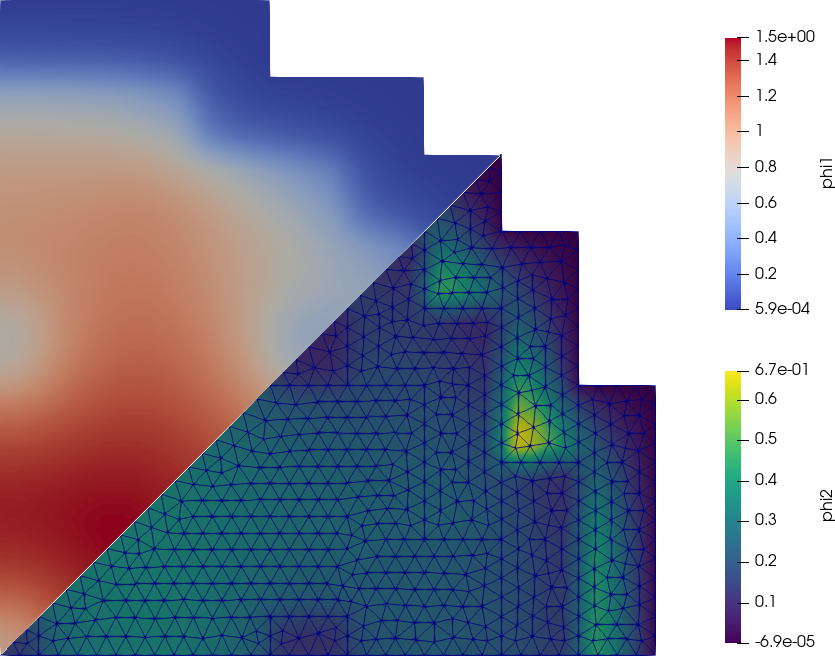Fast and thermal flux for the 2D IAEA PWR benchmark (2021) # 14 Cube-spherical bare reactor It is easy to compute the effective multiplication factor of a one-group bare cubical reactor. Or a spherical reactor. And we know that for the same mass, the k_\text{eff} for the former is smaller than for the latter.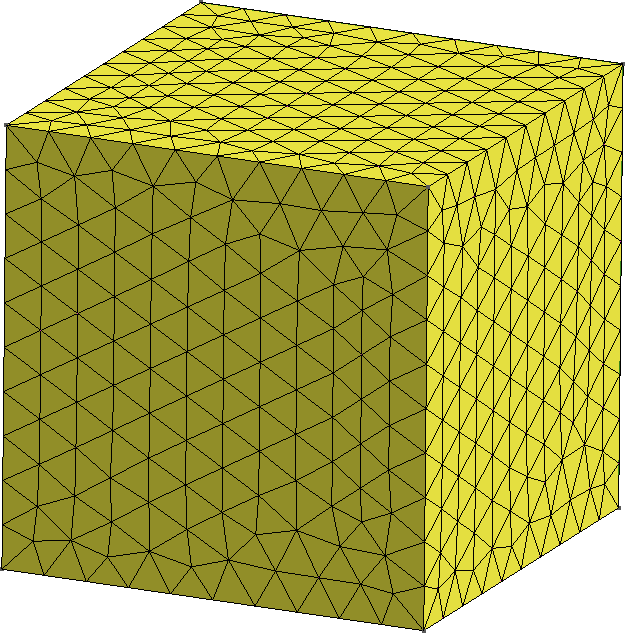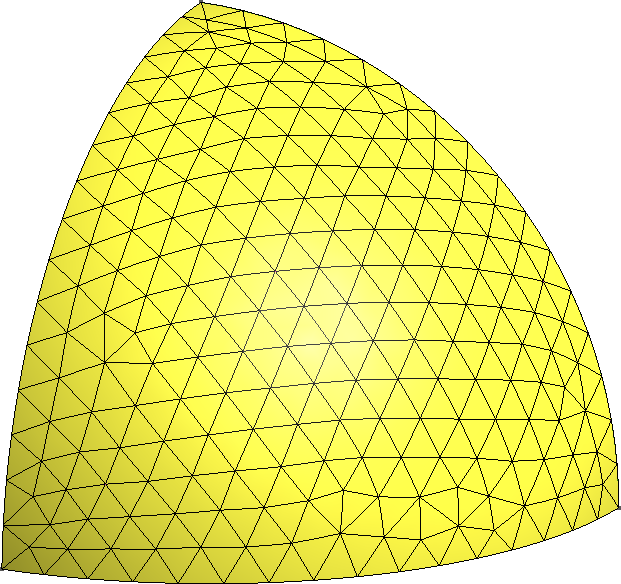Figure 7: One eight of two bare reactors. a — Cubical reactor, b — Spherical reactor But what happens “in the middle”? That is to say, how does k_\text{eff} changes when we morph the cube into a sphere? Enter Gmsh & Feenox.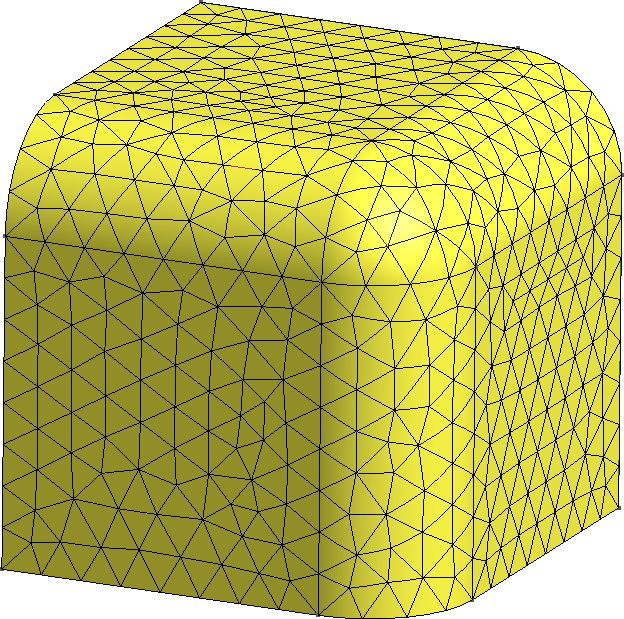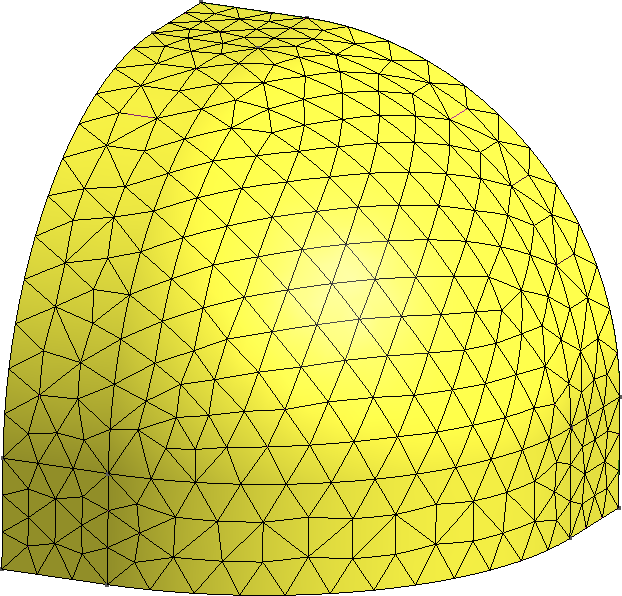Figure 8: Continuous morph between a cube and a sphere. a — 75% cube/25% sphere, b — 50% cube/50% sphere, c — 25% cube/75% sphere import os import math import gmsh def create_mesh(vol, F): gmsh.initialize() gmsh.option.setNumber("General.Terminal", 0) f = 0.01*F a = (vol / (1/8*4/3*math.pi*f**3 + 3*1/4*math.pi*f**2*(1-f) + 3*f*(1-f)**2 + (1-f)**3))**(1.0/3.0) internal = [] gmsh.model.add("cubesphere") if (F < 1): # a cube gmsh.model.occ.addBox(0, 0, 0, a, a, a, 1) internal = [1,3,5] external = [2,4,6] elif (F > 99): # a sphere gmsh.model.occ.addSphere(0, 0, 0, a, 1, 0, math.pi/2, math.pi/2) internal = [2,3,4] external =  else: gmsh.model.occ.addBox(0, 0, 0, a, a, a, 1) gmsh.model.occ.fillet(, [12, 7, 6], [f*a], True) internal = [1,4,6] external = [2,3,5,7,8,9,10] gmsh.model.occ.synchronize() gmsh.model.addPhysicalGroup(3, , 1) gmsh.model.setPhysicalName(3, 1, "fuel") gmsh.model.addPhysicalGroup(2, internal, 2) gmsh.model.setPhysicalName(2, 2, "internal") gmsh.model.addPhysicalGroup(2, external, 3) gmsh.model.setPhysicalName(2, 3, "external") gmsh.model.occ.synchronize() gmsh.option.setNumber("Mesh.ElementOrder", 2) gmsh.option.setNumber("Mesh.Optimize", 1) gmsh.option.setNumber("Mesh.OptimizeNetgen", 1) gmsh.option.setNumber("Mesh.HighOrderOptimize", 1) gmsh.option.setNumber("Mesh.CharacteristicLengthMin", a/10); gmsh.option.setNumber("Mesh.CharacteristicLengthMax", a/10); gmsh.model.mesh.generate(3) gmsh.write("cubesphere-%g.msh"%(F)) gmsh.model.remove() #gmsh.fltk.run() gmsh.finalize() return def main(): vol0 = 100**3 for F in range(0,101,5): # mesh refinement level create_mesh(vol0, F) # TODO: FeenoX Python API! os.system("feenox cubesphere.fee %g"%(F)) if __name__ == "__main__": main() PROBLEM neutron_diffusion DIMENSIONS 3 READ_MESH cubesphere-$1.msh DIMENSIONS 3

# MATERIAL fuel
D1 = 1.03453E+00
Sigma_a1 = 5.59352E-03
nuSigma_f1 = 6.68462E-03
Sigma_s1.1 = 3.94389E-01

PHYSICAL_GROUP fuel DIM 3
BC internal    mirror
BC external    vacuum

SOLVE_PROBLEM

PRINT HEADER $1 keff 1e5*(keff-1)/keff fuel_volume $ python cubesphere.py | tee cubesphere.dat
0       1.05626 5326.13 1e+06
5       1.05638 5337.54 999980
10      1.05675 5370.58 999980
15      1.05734 5423.19 999992
20      1.05812 5492.93 999995
25      1.05906 5576.95 999995
30      1.06013 5672.15 999996
35      1.06129 5775.31 999997
40      1.06251 5883.41 999998
45      1.06376 5993.39 999998
50      1.06499 6102.55 999998
55      1.06619 6208.37 999998
60      1.06733 6308.65 999998
65      1.06839 6401.41 999999
70      1.06935 6485.03 999998
75      1.07018 6557.96 999998
80      1.07088 6618.95 999998
85      1.07143 6666.98 999999
90      1.07183 6701.24 999999
95      1.07206 6721.33 999998
100     1.07213 6727.64 999999
$Static reactivity vs. percentage of sphericity # 15 Parallelepiped whose Young’s modulus is a function of the temperature The thermo-mechanical problem is solved in two stages. First, the heat conduction equation is solved over a coarse first-order mesh to find the non-dimensional temperature distribution. Then, a mechanical problem is solved where T(x,y,z) is read from the first mesh and interpolated into a finer second-order mesh so to as evaluate the non-dimensional Young’s modulus as E\Big(T(x,y,z)\Big) = \frac{1000}{800-T(x,y,z)} Note that there are not thermal expansion effects (i.e. the thermal expansion coefficient is \alpha=0). Yet, suprinsingly enough, the problem has analytical solutions for both the temperature and the displacement fields. References: ## 15.1 Thermal problem The following input solves the thermal problem over a coarse first-order mesh, writes the resulting temperature distribution into parallelepiped-temperature.msh, and prints the L_2 error of the numerical result with respect to the analytical solution T(x,y,z) = 40 - 2x - 3y - 4z. PROBLEM thermal 3D READ_MESH parallelepiped-coarse.msh k = 1 # unitary non-dimensional thermal conductivity # boundary conditions BC left q=+2 BC right q=-2 BC front q=+3 BC back q=-3 BC bottom q=+4 BC top q=-4 BC A T=0 SOLVE_PROBLEM WRITE_MESH parallelepiped-temperature.msh T # compute the L-2 norm of the error in the displacement field Te(x,y,z) = 40 - 2*x - 3*y - 4*z # analytical solution, "e" means exact INTEGRATE (T(x,y,z)-Te(x,y,z))^2 RESULT num PHYSICAL_GROUP bulk DIM 3 # this is just to compute the volume PRINT num/bulk_volume $ gmsh -3 parallelepiped.geo -order 1 -clscale 2 -o parallelepiped-coarse.msh
[...]
Info    : 117 nodes 567 elements
Info    : Writing 'parallelepiped-coarse.msh'...
Info    : Done writing 'parallelepiped-coarse.msh'
Info    : Stopped on Fri Dec 10 10:32:30 2021 (From start: Wall 0.0386516s, CPU 0.183052s)
$feenox parallelepiped-thermal.fee 6.18981e-12$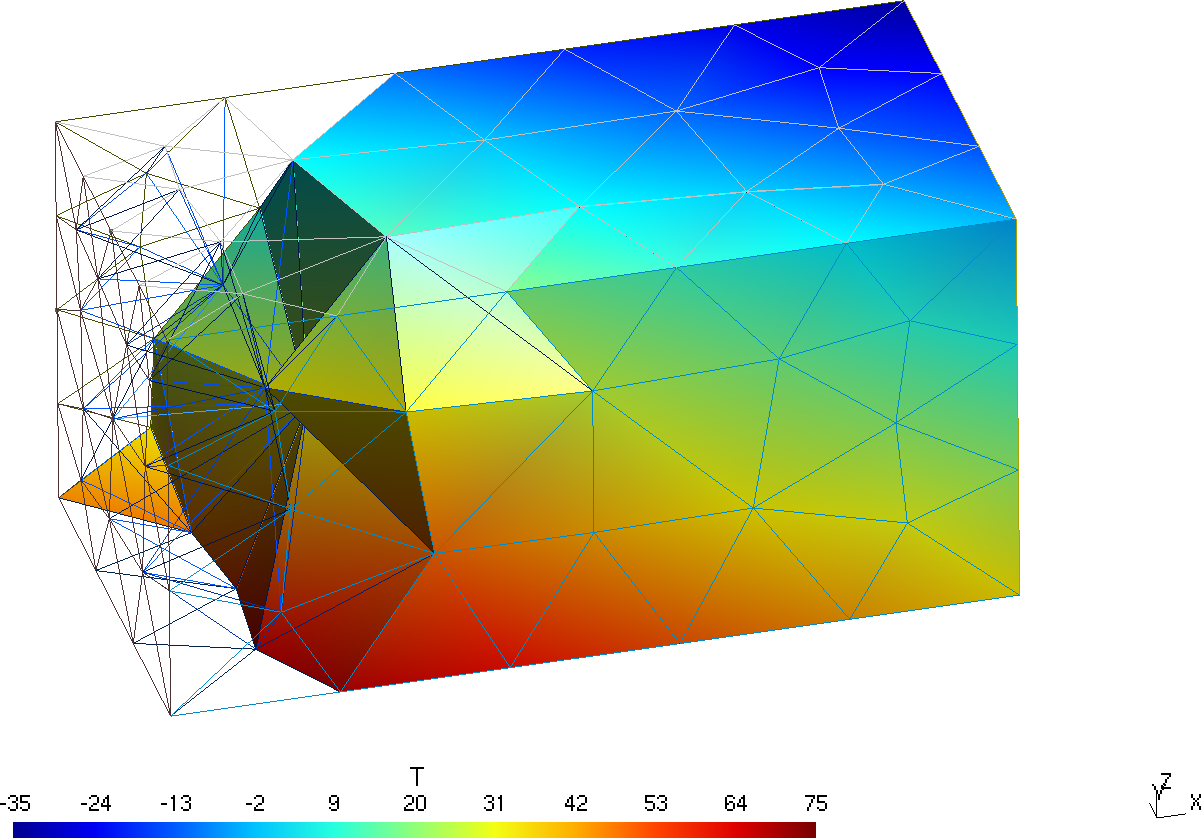Temperature distribution over the coarse mesh in Gmsh (yes, it is a rainbow pallete)

## 15.2 Mechanical problem

Now this input file reads the scalar function T stored in the coarse first-order mesh file parallelepiped-temperature.msh and uses it to solve the mechanical problem in the finer second-order mesh parallelepiped.msh. The numerical solution for the displacemetns over the fine mesh is written in a VTK file (along with the temperature as interpolated from the coarse mesh) and compared to the analytical solution using the L_2 norm.

PROBLEM mechanical 3D

# this is where we solve the mechanical problem

# this is where we read the temperature from

# mechanical properties
E(x,y,z) = 1000/(800-T(x,y,z))   # young's modulus
nu = 0.3                         # poisson's ratio

# boundary conditions
BC O fixed
BC B u=0 w=0
BC C u=0

# here "load" is a fantasy name applied to both "left" and "right"
BC load tension=1 PHYSICAL_GROUP left PHYSICAL_GROUP right

SOLVE_PROBLEM
WRITE_MESH parallelepiped-mechanical.vtk T VECTOR u v w

# analytical solutions
h = 10
A = 0.002
B = 0.003
C = 0.004
D = 0.76

# the "e" means exact
ue(x,y,z) := A/2*(x^2 + nu*(y^2+z^2)) + B*x*y + C*x*z + D*x - nu*A*h/4*(y+z)
ve(x,y,z) := -nu*(A*x*y + B/2*(y^2-z^2+x^2/nu) + C*y*z + D*y -A*h/4*x - C*h/4*z)
we(x,y,z) := -nu*(A*x*z + B*y*z + C/2*(z^2-y^2+x^2/nu) + D*z + C*h/4*y - A*h/4*x)

# compute the L-2 norm of the error in the displacement field
INTEGRATE (u(x,y,z)-ue(x,y,z))^2+(v(x,y,z)-ve(x,y,z))^2+(w(x,y,z)-we(x,y,z))^2 RESULT num MESH parallelepiped.msh
INTEGRATE 1 RESULT den MESH parallelepiped.msh
PRINT num/den
$gmsh -3 parallelepiped.geo -order 2 [...] Info : 2564 nodes 2162 elements Info : Writing 'parallelepiped.msh'... Info : Done writing 'parallelepiped.msh' Info : Stopped on Fri Dec 10 10:39:27 2021 (From start: Wall 0.165707s, CPU 0.258751s)$ feenox parallelepiped-mechanical.fee
0.000345839
$Displacements and temperature distribution over the fine mesh in Paraview (yes, still a rainbow pallete) # 16 Non-dimensional transient heat conduction on a cylinder Let us solve a dimensionless transient problem over a cylinder. Conductivity and heat capacity are unity. Initial condition is a linear temperature profile along the x axis: T(x,y,z,0) = x The base of the cylinder has a prescribed time and space-dependent temperature T(0,y,z,t) = \sin( 2\pi \cdot t) \cdot \sin( 2\pi \cdot y) The other faces have a convection conditions with (non-dimensional) heat transfer coefficient h=0.1 and T_\text{ref} = 1.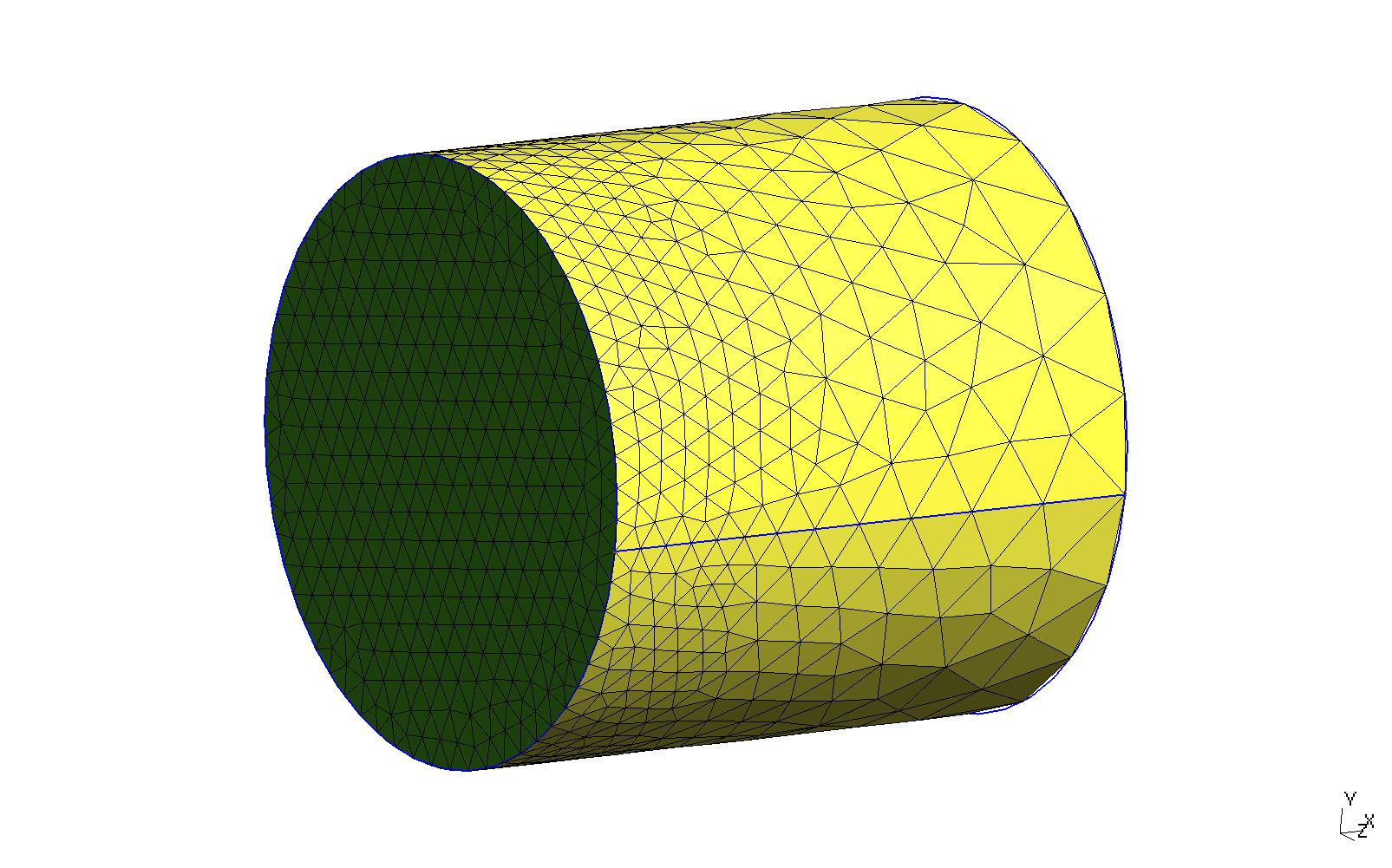Locally-refined cylinder for a transient thermal problem. PROBLEM thermal 3D READ_MESH cylinder.msh end_time = 2 # final time [ non-dimensional units ] # the time step is automatically computed # initial condition (if not given, stead-state is computed) T_0(x,y,z) = x # dimensionless uniform and constant material properties k = 1 kappa = 1 # BCs BC hot T=sin(2*pi*t)*sin(2*pi*y) BC cool h=0.1 Tref=1 SOLVE_PROBLEM # print the temperature at the center of the base vs time PRINT %e t T(0,0,0) T(0.5,0,0) T(1,0,0) WRITE_MESH temp-cylinder.msh T IF done PRINT "\# open temp-anim-cylinder.geo in Gmsh to create a quick rough video" PRINT "\# run temp-anim-cylinder.py to get a nicer and smoother video" ENDIF $ gmsh -3 cylinder.geo
[...]
Info    : Done optimizing mesh (Wall 0.624941s, CPU 0.624932s)
Info    : 1986 nodes 10705 elements
Info    : Writing 'cylinder.msh'...
Info    : Done writing 'cylinder.msh'
Info    : Stopped on Fri Dec 24 10:35:32 2021 (From start: Wall 0.800542s, CPU 0.896698s)
$feenox temp-cylinder-tran.fee 0.000000e+00 0.000000e+00 5.000000e-01 1.000000e+00 1.451938e-04 4.406425e-07 5.000094e-01 9.960851e-01 3.016938e-04 9.155974e-07 5.000171e-01 9.921274e-01 5.566768e-04 1.689432e-06 5.000251e-01 9.862244e-01 8.565589e-04 2.599523e-06 5.000292e-01 9.800113e-01 1.245867e-03 3.780993e-06 5.000280e-01 9.728705e-01 1.780756e-03 5.404230e-06 5.000176e-01 9.643259e-01 2.492280e-03 7.563410e-06 4.999932e-01 9.545723e-01 3.428621e-03 1.040457e-05 4.999538e-01 9.436480e-01 [...] 1.978669e+00 -6.454358e-05 1.500891e-01 2.286112e-01 1.989334e+00 -3.234439e-05 1.500723e-01 2.285660e-01 2.000000e+00 1.001730e-14 1.500572e-01 2.285223e-01 # open temp-anim-cylinder.geo in Gmsh to create a quick rough video # run temp-anim-cylinder.py to get a nicer and smoother video$ python3 temp-anim-cylinder.py
Info    : 1986 nodes
Info    : 10612 elements
0 1 0.0
0.01 12 0.8208905327853042
0.02 15 0.8187351216040447
0.03 17 0.7902629708599855
[...]
Info    : Writing 'temp-cylinder-smooth-198.png'...
Info    : Done writing 'temp-cylinder-smooth-198.png'
199
Info    : Writing 'temp-cylinder-smooth-199.png'...
Info    : Done writing 'temp-cylinder-smooth-199.png'
all frames dumped, now run
ffmpeg -framerate 20 -f image2 -i temp-cylinder-smooth-%03d.png temp-cylinder-smooth.mp4
to get a video
$ffmpeg -y -f image2 -i temp-cylinder-smooth-%03d.png -framerate 20 -pix_fmt yuv420p -c:v libx264 -filter:v crop='floor(in_w/2)*2:floor(in_h/2)*2' temp-cylinder-smooth.mp4 [...]$

# 17 Five natural modes of a cantilevered wire

Back in college, we had this subject Experimental Physics 101. I had to measure the natual modes of two cantilevered wires and determine the Young modulus of of those measurements. The report is here. Two comments: 1. It is in Spanish 2. There was a systematic error and a factor of two sneaked in into the measured values

Here is a finite-element version of the experimental setup with a comparison to then theoretical values written directly as Markdown tables. The material (either aluminum or copper) and the mesh type (either tet or hex) and be chosen at runtime through command line arguments.

#
DEFAULT_ARGUMENT_VALUE 1 hex       # mesh, either hex or unstruct
DEFAULT_ARGUMENT_VALUE 2 copper    # material, either copper or aluminum

l = 0.5*303e-3   # cantilever wire length [ m ]
d = 1.948e-3     # wire diameter [ m ]

# material properties for copper
m_copper = 0.5*8.02e-3  # total mass (half the measured because of the experimental disposition) [ kg ]
E_copper = 2*66.2e9     # [ Pa ] Young modulus (twice because the factor-two error)

# material properties for aluminum
m_aluminum = 0.5*2.67e-3
E_aluminum = 2*40.2e9

# problem’s properties
E = E_$2 # [ MPa ] rho = m_$2/(pi*(0.5*d)^2*l)  # [ kg / m^3 ] density = mass (measured) / volume
nu = 0                       # Poisson’s ratio (does not appear in Euler-Bernoulli)

# analytical solution
VECTOR kl
VECTOR f_euler

# first compute the first five roots ok cosh(kl)*cos(kl)+1
kl[i] = root(cosh(t)*cos(t)+1, t, 3*i-2,3*i+1)

# then compute the frequencies according to Euler-Bernoulli
# note that we need to use SI inside the square root
A = pi * (d/2)^2
I = pi/4 * (d/2)^4
f_euler[i] = 1/(2*pi) * kl(i)^2 * sqrt((E * I)/(rho * A * l^4))

# now compute the modes numerically with FEM
# note that each mode is duplicated as it is degenerated
READ_MESH wire-$1.msh DIMENSIONS 3 PROBLEM modal MODES 10 BC fixed fixed SOLVE_PROBLEM # github-formatted markdown table # compare the frequencies PRINT " \$n\$| FEM [Hz] | Euler [Hz] | Relative difference [%]" PRINT ":------:|:-------------:|:-------------:|:--------------:" PRINT_VECTOR SEP "\t|\t" i %.4g f(2*i-1) f_euler %.2f 100*(f_euler(i)-f(2*i-1))/f_euler(i) PRINT PRINT ":$2 wire over $1 mesh" # commonmark table PRINT PRINT " \$n\$| \$L\$| \$\\Gamma\$| \$\\mu\$| \$M\$" PRINT ":------:+:---------------------:+:---------------------:+:-------------:+:--------------:" PRINT_VECTOR SEP "\t|\t" i "%+.1e" L Gamma "%.4f" mu Mu PRINT PRINT ":$2 wire over $1 mesh, participation and excitation factors \$L\$and \$\\Gamma\$, effective per-mode and cummulative mass fractions \$\\mu\$and \$M\$" # write the modes into a vtk file WRITE_MESH wire-$1-$2.vtk \ VECTOR u1 v1 w1 VECTOR u2 v2 w2 VECTOR u3 v3 w3 \ VECTOR u4 v4 w4 VECTOR u5 v5 w5 VECTOR u6 v6 w6 \ VECTOR u7 v7 w7 VECTOR u8 v8 w8 VECTOR u9 v9 w9 VECTOR u10 v10 w10 # and into a msh file WRITE_MESH wire-$1-$2.msh { u1 v1 w1 u2 v2 w2 u3 v3 w3 u4 v4 w4 u5 v5 w5 u6 v6 w6 u7 v7 w7 u8 v8 w8 u9 v9 w9 u10 v10 w10 } $ gmsh -3 wire-hex.geo
[...]
Info    : Done meshing order 2 (Wall 0.0169025s, CPU 0.016804s)
Info    : 8398 nodes 4676 elements
Info    : Writing 'wire-hex.msh'...
Info    : Done writing 'wire-hex.msh'
Info    : Stopped on Fri Dec 24 17:07:19 2021 (From start: Wall 0.0464517s, CPU 0.133498s)
$gmsh -3 wire-tet.geo [...] Info : Done optimizing mesh (Wall 0.0229018s, CPU 0.022892s) Info : 16579 nodes 13610 elements Info : Writing 'wire-tet.msh'... Info : Done writing 'wire-tet.msh' Info : Stopped on Fri Dec 24 17:07:59 2021 (From start: Wall 2.5798s, CPU 2.64745s)$ feenox wire.fee
$n$   |    FEM [Hz]   |   Euler [Hz]  |  Relative difference [%]
:------:|:-------------:|:-------------:|:--------------:
1       |       45.84   |       45.84   |       0.02
2       |       287.1   |       287.3   |       0.06
3       |       803.4   |       804.5   |       0.13
4       |       1573    |       1576    |       0.24
5       |       2596    |       2606    |       0.38

: copper wire over hex mesh

$n$   |          $L$          |       $\Gamma$        |      $\mu$    |       $M$
:------:+:---------------------:+:---------------------:+:-------------:+:--------------:
1       |       +1.3e-03        |       +4.2e-01        |       0.1371  |       0.1371
2       |       -1.8e-03        |       -5.9e-01        |       0.2716  |       0.4087
3       |       +9.1e-05        |       +1.7e-02        |       0.0004  |       0.4091
4       |       -1.7e-03        |       -3.0e-01        |       0.1252  |       0.5343
5       |       -3.3e-05        |       -5.9e-03        |       0.0000  |       0.5343
6       |       -9.9e-04        |       -1.8e-01        |       0.0431  |       0.5775
7       |       +7.3e-04        |       +1.2e-01        |       0.0221  |       0.5995
8       |       +4.5e-06        |       +7.5e-04        |       0.0000  |       0.5995
9       |       +5.4e-04        |       +9.9e-02        |       0.0134  |       0.6129
10      |       +2.7e-05        |       +4.9e-03        |       0.0000  |       0.6129

: copper wire over hex mesh, participation and excitation factors $L$ and $\Gamma$, effective per-mode and cummulative mass fractions $\mu$ and $M$
$feenox wire.fee hex copper | pandoc -o wire-hex-copper.html$ feenox wire.fee tet copper   | pandoc -o wire-tet-copper.html
$feenox wire.fee hex aluminum | pandoc -o wire-hex-aluminum.html$ feenox wire.fee tet aluminum | pandoc -o wire-tet-aluminum.html
copper wire over hex mesh
n FEM [Hz] Euler [Hz] Relative difference [%]
1 45.84 45.84 0.02
2 287.1 287.3 0.06
3 803.4 804.5 0.13
4 1573 1576 0.24
5 2596 2606 0.38
copper wire over hex mesh, participation and excitation factors L and \Gamma, effective per-mode and cummulative mass fractions \mu and M
n L \Gamma \mu M
1 -1.8e-03 -5.9e-01 0.2713 0.2713
2 +1.3e-03 +4.2e-01 0.1374 0.4087
3 +9.7e-05 +1.8e-02 0.0004 0.4091
4 -1.6e-03 -3.1e-01 0.1251 0.5343
5 -3.5e-05 -6.3e-03 0.0001 0.5343
6 -9.9e-04 -1.8e-01 0.0431 0.5774
7 +7.2e-04 +1.2e-01 0.0221 0.5995
8 -8.6e-06 -1.5e-03 0.0000 0.5995
9 -2.6e-05 -4.7e-03 0.0000 0.5996
10 +5.4e-04 +9.9e-02 0.0134 0.6130
copper wire over tet mesh
n FEM [Hz] Euler [Hz] Relative difference [%]
1 45.84 45.84 0.00
2 287.2 287.3 0.05
3 803.4 804.5 0.13
4 1573 1576 0.24
5 2596 2606 0.38
copper wire over tet mesh, participation and excitation factors L and \Gamma, effective per-mode and cummulative mass fractions \mu and M
n L \Gamma \mu M
1 -1.9e-03 -6.1e-01 0.2925 0.2925
2 +1.2e-03 +3.8e-01 0.1163 0.4087
3 -1.0e-03 -3.3e-01 0.0861 0.4948
4 +7.0e-04 +2.3e-01 0.0395 0.5343
5 -6.0e-04 -1.9e-01 0.0292 0.5634
6 +4.2e-04 +1.3e-01 0.0140 0.5774
7 -4.0e-04 -1.3e-01 0.0133 0.5908
8 +3.3e-04 +1.1e-01 0.0087 0.5995
9 +3.5e-04 +1.1e-01 0.0096 0.6091
10 -2.2e-04 -6.9e-02 0.0038 0.6129
aluminum wire over hex mesh
n FEM [Hz] Euler [Hz] Relative difference [%]
1 61.91 61.92 0.02
2 387.8 388 0.06
3 1085 1086 0.13
4 2124 2129 0.24
5 3506 3519 0.38
aluminum wire over hex mesh, participation and excitation factors L and \Gamma, effective per-mode and cummulative mass fractions \mu and M
n L \Gamma \mu M
1 -6.9e-04 -6.2e-01 0.3211 0.3211
2 +3.6e-04 +3.3e-01 0.0876 0.4087
3 +4.2e-05 +2.4e-02 0.0008 0.4095
4 -5.4e-04 -3.1e-01 0.1248 0.5343
5 +3.7e-05 +2.3e-02 0.0006 0.5349
6 -3.0e-04 -1.9e-01 0.0425 0.5774
7 +2.4e-04 +1.2e-01 0.0221 0.5995
8 -3.2e-06 -1.6e-03 0.0000 0.5995
9 +1.8e-04 +9.8e-02 0.0132 0.6127
10 -9.5e-06 -5.2e-03 0.0000 0.6128
aluminum wire over tet mesh
n FEM [Hz] Euler [Hz] Relative difference [%]
1 61.91 61.92 0.00
2 387.8 388 0.05
3 1085 1086 0.13
4 2124 2129 0.24
5 3506 3519 0.38
aluminum wire over tet mesh, participation and excitation factors L and \Gamma, effective per-mode and cummulative mass fractions \mu and M
n L \Gamma \mu M
1 -6.4e-04 -6.1e-01 0.2923 0.2923
2 +4.0e-04 +3.8e-01 0.1164 0.4087
3 -3.5e-04 -3.3e-01 0.0861 0.4948
4 +2.3e-04 +2.3e-01 0.0395 0.5343
5 -2.0e-04 -1.9e-01 0.0292 0.5634
6 +1.4e-04 +1.3e-01 0.0140 0.5774
7 -1.3e-04 -1.3e-01 0.0133 0.5908
8 +1.1e-04 +1.1e-01 0.0087 0.5995
9 +1.2e-04 +1.1e-01 0.0096 0.6091
10 -7.3e-05 -6.9e-02 0.0038 0.6129

# 18 On the evaluation of thermal expansion coefficients

When solving thermal-mechanical problems it is customary to use thermal expansion coefficients in order to take into account the mechanical strains induced by changes in the material temperature with respect to a reference temperature where the deformation is identically zero. These coefficients \alpha are defined as the partial derivative of the strain \epsilon with respect to temperature T such that differential relationships like

d\epsilon = \frac{\partial \epsilon}{\partial T} \, dT = \alpha \cdot dT

hold. This derivative \alpha is called the instantaneous thermal expansion coefficient. For finite temperature increments, one would like to be able to write

\Delta \epsilon = \alpha \cdot \Delta T

But if the strain is not linear with respect to the temperature—which is the most common case—then \alpha depends on T. Therefore, when dealing with finite temperature increments \Delta T = T-T_0 where the thermal expansion coefficient \alpha(T) depends on the temperature itself then mean values for the thermal expansion ought to be used:

\Delta \epsilon = \int_{\epsilon_0}^{\epsilon} d\epsilon^{\prime} = \int_{T_0}^{T} \frac{\partial \epsilon}{\partial T^\prime} \, dT^\prime = \int_{T_0}^{T} \alpha(T^\prime) \, dT^\prime

We can multiply and divide by \Delta T to obtain

\int_{T_0}^{T} \alpha(T^\prime) \, dT^\prime \cdot \frac{\Delta T}{\Delta T} = \bar{\alpha}(T) \cdot \Delta T

where the mean expansion coefficient for the temperature range [T_0,T] is

\bar{\alpha}(T) = \frac{\displaystyle \int_{T_0}^{T} \alpha(T^\prime) \, dT^\prime}{T-T_0}

This is of course the classical calculus result of the mean value of a continuous one-dimensional function in a certain range.

Let \epsilon(T) be the linear thermal expansion of a given material in a certain direction when heating a piece of such material from an initial temperature T_0 up to T so that \epsilon(T_0)=0.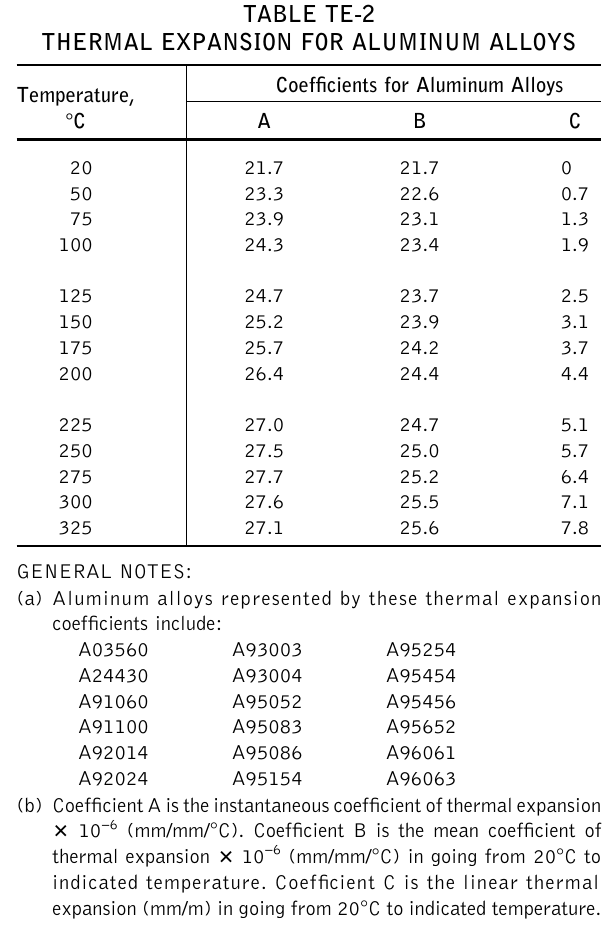Figure 9: Table TE2 of thermal expansion properties for Aluminum alloys from ASME II Part D (figure from this report.

From our previous analysis, we can see that in fig. 9:

\begin{aligned} A(T) &= \alpha(T) = \frac{\partial \epsilon}{\partial T} \\ B(T) &= \bar{\alpha}(T) = \frac{\epsilon(T)}{T-T_0} = \frac{\displaystyle \int_{T_0}^{T} \alpha(T^\prime) \, dT^\prime}{T - T_0} \\ C(T) &= \epsilon(T) = \int_{T_0}^T \alpha(T^\prime) \, dT^\prime \end{aligned}

Therefore,

1. A(T) can be computed out of C(T)
2. B(T) can be computed either out of A(T) or C(T)
3. C(T) can be computed out of A(T)
# just in case we wanted to interpolate with another method (linear, splines, etc.)
DEFAULT_ARGUMENT_VALUE 1 steffen

# read columns from data file and interpolate
# A is the instantaenous coefficient of thermal expansion x 10^-6 (mm/mm/ºC)
FUNCTION A(T) FILE asme-expansion-table.dat COLUMNS 1 2 INTERPOLATION $1 # B is the mean coefficient of thermal expansion x 10^-6 (mm/mm/ºC) in going # from 20ºC to indicated temperature FUNCTION B(T) FILE asme-expansion-table.dat COLUMNS 1 3 INTERPOLATION$1
# C is the linear thermal expansion (mm/m) in going from 20ºC
# to indicated temperature
FUNCTION C(T) FILE asme-expansion-table.dat COLUMNS 1 4 INTERPOLATION $1 VAR T' # dummy variable for integration T0 = 20 # reference temperature T_min = vecmin(vec_A_T) # smallest argument of function A(T) T_max = vecmax(vec_A_T) # largest argument of function A(T) # compute one column from another one A_fromC(T) := 1e3*derivative(C(T'), T', T) B_fromA(T) := integral(A(T'), T', T0, T)/(T-T0) B_fromC(T) := 1e3*C(T)/(T-T0) # C is in mm/m, hence the 1e3 C_fromA(T) := 1e-3*integral(A(T'), T', T0, T) # write interpolated results PRINT_FUNCTION A A_fromC B B_fromA B_fromC C C_fromA MIN T_min+1 MAX T_max-1 STEP 1 $ cat asme-expansion-table.dat
# temp  A       B        C
20      21.7    21.7     0
50      23.3    22.6     0.7
75      23.9    23.1     1.3
100     24.3    23.4     1.9
125     24.7    23.7     2.5
150     25.2    23.9     3.1
175     25.7    24.2     3.7
200     26.4    24.4     4.4
225     27.0    24.7     5.1
250     27.5    25.0     5.7
275     27.7    25.2     6.4
300     27.6    25.5     7.1
325     27.1    25.6     7.8
$feenox asme-expansion.fee > asme-expansion-interpolation.dat$ pyxplot asme-expansion.ppl
$The conclusion (see this, this and this reports) is that values rounded to only one decimal value as presented in the ASME code section II subsection D tables are not enough to satisfy the mathematical relationships between the physical magnitudes related to thermal expansion properties of the materials listed. Therefore, care has to be taken as which of the three columns is chosen when using the data for actual thermo-mechanical numerical computations. As an exercise, the reader is encouraged to try different interpolation algorithms to see how the results change. Spoiler alert: they are also highly sensible to the interpolation method used to “fill in” the gaps between the table values. ## 18.1 Orthotropic free expansion of a cube To illustrate the point of the previous discussion, let us solve the thermal expansion of an unrestrained unitary cube [0,1~\text{mm}]\times[0,1~\text{mm}]\times[0,1~\text{mm}] subject to a linear radially-symmetric temperature field T(x,y,z) = 30 \text{ºC} + 150 \frac{\text{ºC}}{\text{mm}} \sqrt{x^2+y^2+z^2} with a mean thermal expansion coefficient for each of the three directions x, y and z computed from each of the three columns of the ASME table TE-2, respectively. If the data was consistent, the displacement at any point with the same coordinates x=y=z would be exactly equal. DEFAULT_ARGUMENT_VALUE 1 steffen DEFAULT_ARGUMENT_VALUE 2 hex PROBLEM mechanical READ_MESH cube-hex.msh # aluminum-like linear isotropic material properties E = 69e3 nu = 0.28 # free expansion BC left u=0 BC front v=0 BC bottom w=0 # reference temperature is 20ºC T0 = 20 # spatial temperature distribution symmetric wrt x,y & z T(x,y,z) = 30+150*sqrt(x^2+y^2+z^2) # read ASME data FUNCTION A(T') FILE asme-expansion-table.dat COLUMNS 1 2 INTERPOLATION$1
FUNCTION B(T') FILE asme-expansion-table.dat COLUMNS 1 3 INTERPOLATION $1 FUNCTION C(T') FILE asme-expansion-table.dat COLUMNS 1 4 INTERPOLATION$1

# remember that the thermal expansion coefficients have to be
#  1. the mean value between T0 and T
#  2. functions of space, so temperature has to be written as T(x,y,z)

# in the x direction, we use column B directly
alpha_x(x,y,z) = 1e-6*B(T(x,y,z))

# in the y direction, we convert column A to mean
alpha_y(x,y,z) = 1e-6*integral(A(T'), T', T0, T(x,y,z))/(T(x,y,z)-T0)

# in the z direction, we convert column C to mean
alpha_z(x,y,z) = 1e-3*C(T(x,y,z))/(T(x,y,z)-T0)

SOLVE_PROBLEM

WRITE_MESH cube-orthotropic-expansion-$1-$2.vtk T VECTOR u v w
PRINT %.3e "displacement in x at (1,1,1) = " u(1,1,1)
PRINT %.3e "displacement in y at (1,1,1) = " v(1,1,1)
PRINT %.3e "displacement in z at (1,1,1) = " w(1,1,1)
$gmsh -3 cube-hex.geo [...]$ gmsh -3 cube-tet.geo
[...]
$feenox cube-orthotropic-expansion.fee displacement in x at (1,1,1) = 4.451e-03 displacement in y at (1,1,1) = 4.449e-03 displacement in z at (1,1,1) = 4.437e-03$ feenox cube-orthotropic-expansion.fee linear tet
displacement in x at (1,1,1) =  4.451e-03
displacement in y at (1,1,1) =  4.447e-03
displacement in z at (1,1,1) =  4.438e-03
$feenox cube-orthotropic-expansion.fee akima hex displacement in x at (1,1,1) = 4.451e-03 displacement in y at (1,1,1) = 4.451e-03 displacement in z at (1,1,1) = 4.437e-03$ feenox cube-orthotropic-expansion.fee splines tet
displacement in x at (1,1,1) =  4.451e-03
displacement in y at (1,1,1) =  4.450e-03
displacement in z at (1,1,1) =  4.438e-03
$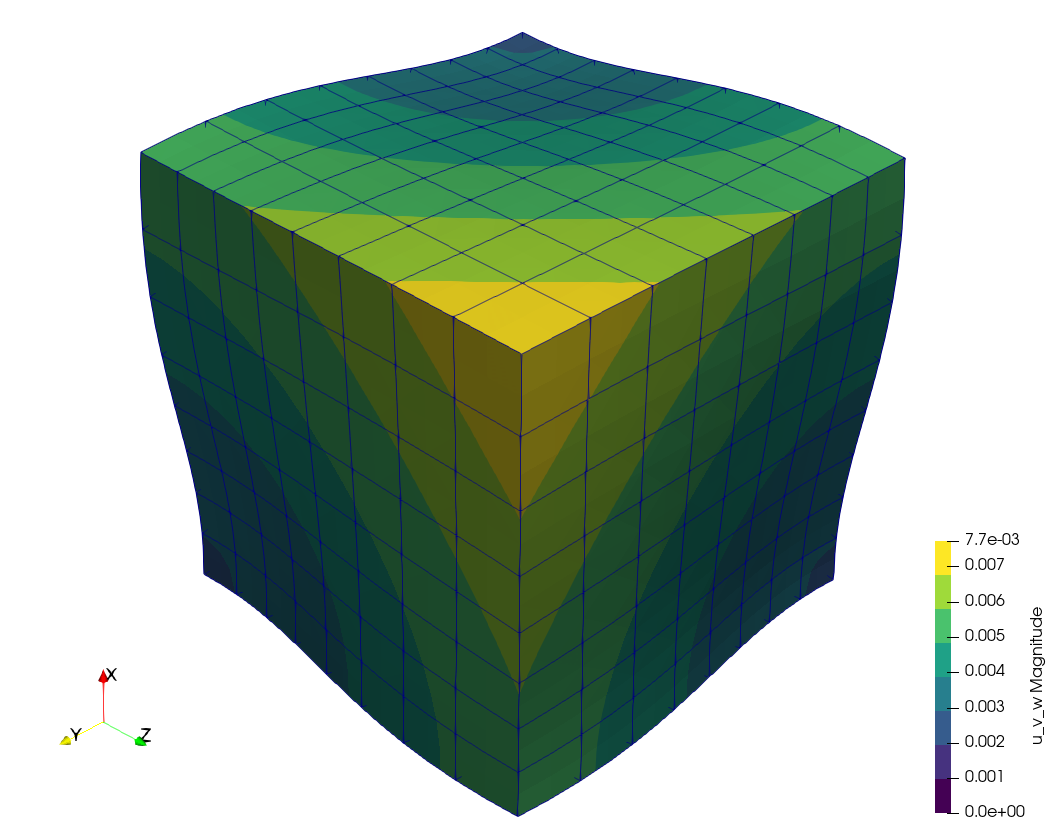Warped displacement (\times 500) of the cube using ASME’s three columns. Differences cannot be seen graphically, but they are there as the terminal mimic illustrates. Yet, they are not as large nor as sensible to meshing and interpolation settings as one would have expected after seeing the plots from the previous section. # 19 Thermo-elastic expansion of finite cylinders Let us solve the following problem introduced by J. Veeder in his technical report AECL-2660 from 1967.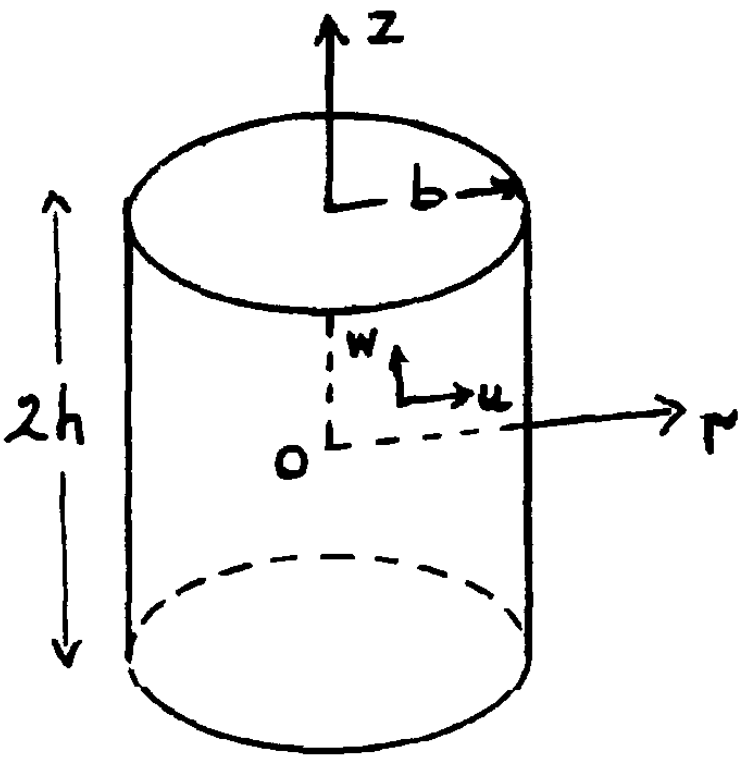Consider a finite solid cylinder (see insert) of radius b and length 2h, with the origin of coordinates at the centre. It may be shown that the temperature distribution in a cylindrical fuel pellet operating in a reactor is given approximately by T(r) = T_0 + T_1 \cdot \left[ 1 - \left(\frac{r}{b} \right)^2 \right] where T_0 is the pellet surface temperature and T_1 is the temperature difference between the centre and surface. The thermal expansion is thus seen to be the sum of two terms, the first of which produces uniform expansion (zero stress) at constant temperature T_0, and is therefore computationally trivial. Tho second term introduces non-uniform body forces which distort the pellet from its original cylindrical shape. The problem is axisymmetric on the azimutal angle and axially-symmetric along the mid-plane. The FeenoX input uses the tangential and radial boundary conditions applied to the base of the upper half of a 3D cylinder. The geometry is meshed using 27-noded hexahedra. Two one-dimensional profiles for the non-dimensional range [0:1] at the external surfaces are written into an ASCII file ready to be plotted: 1. axial dependency of the displacement v(z') = v(0,v,z'h) in the y direction at fixed x=0 and y=b, and 2. radial dependency of the displacement w(r') = w(0,r'b, h) in the z direction at fixed x=0 and z=h These two profiles are compared to the power expansion series given in the original report from 1967. Note that the authors expect a 5% difference between the reference solution and the real one.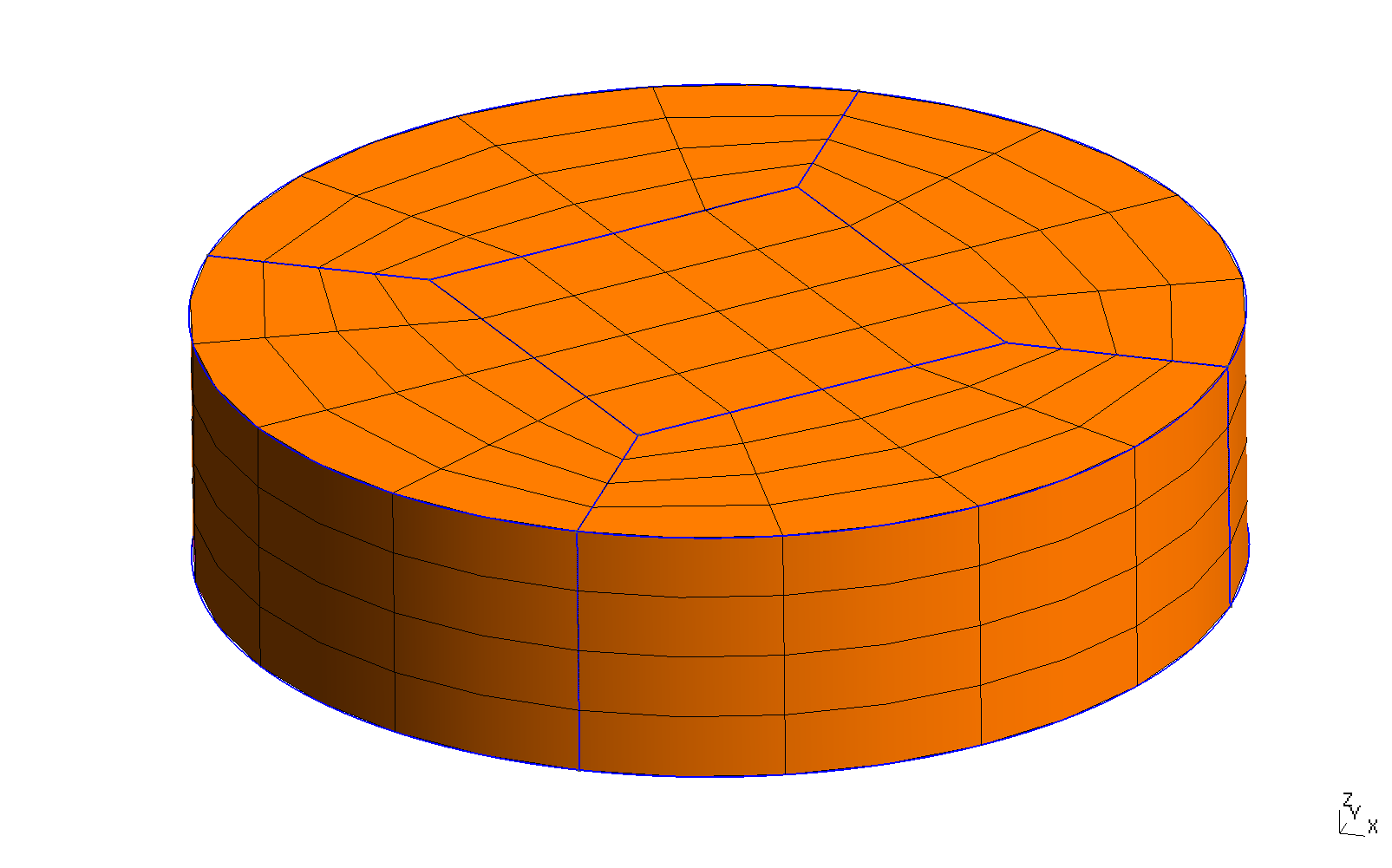3D mesh of the upper half of the Veeder problem PROBLEM mechanical READ_MESH veeder.msh b = 1 # cylinder radius h = 0.5 # cylinder height E = 1 # young modulus (does not matter for the displacement, only for stresses) nu = 1/3 # poisson ratio alpha = 1e-5 # temperature expansion coefficient # temperature distribution as in the original paper T1 = 1 # maximum temperature T0 = 0 # reference temperature (where expansion is zero) T(x,y,z) := T0 + T1*(1-(x^2+y^2)/(b^2)) # boundary conditions (note that the cylinder can still expand on the x-y plane) BC inf tangential radial # solve! SOLVE_PROBLEM # write vtk output WRITE_MESH veeder.vtk T sigma dudx dudy dudz dvdx dvdy dvdz dwdx dwdy dwdz sigma1 sigma2 sigma3 VECTOR u v w # non-dimensional numerical displacement profiles v_num(z') = v(0, b, z'*h)/(alpha*T1*b) w_num(r') = w(0, r'*b, h)/(alpha*T1*b) ######## # reference solution # coefficients of displacement functions for h/b = 0.5 a00 = 0.66056 a01 = -0.44037 a10 = 0.23356 a02 = -0.06945 a11 = -0.10417 a20 = 0.00288 b00 = -0.01773 b01 = -0.46713 b10 = -0.04618 b02 = +0.10417 b11 = -0.01152 b20 = -0.00086 # coefficients of displacement functions for h/b = 1.0 # a00 = 0.73197 # a01 = -0.48798 # a10 = 0.45680 # a02 = -0.01140 # a11 = -0.06841 # a20 = 0.13611 # # b00 = 0.26941 # b01 = -0.45680 # b10 = -0.25670 # b02 = 0.03420 # b11 = -0.27222 # b20 = -0.08167 R(r') = r'^2 - 1 Z(z') = z'^2 - 1 v_ref(r',z') = r' * (a00 + a01*R(r') + a10*Z(z') + a02* R(r')^2 + a11 * R(r')*Z(z') + a20 * Z(z')^2) w_ref(r',z') = z' * (b00 + b01*R(r') + b10*Z(z') + b02* R(r')^2 + b11 * R(r')*Z(z') + b20 * Z(z')^2) PRINT_FUNCTION FILE veeder_v.dat v_num v_ref(1,z') MIN 0 MAX 1 NSTEPS 50 HEADER PRINT_FUNCTION FILE veeder_w.dat w_num w_ref(r',1) MIN 0 MAX 1 NSTEPS 50 HEADER $ gmsh -3 veeder.geo
[...]
$feenox veeder.fee$ pyxplot veeder.ppl
\$